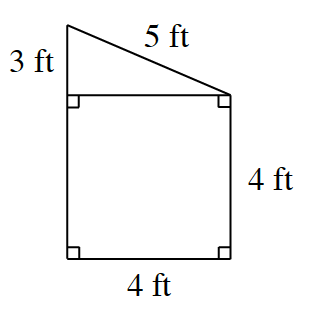### Home > CC2 > Chapter 7 > Lesson 7.1.1 > Problem7-15

7-15.

Find the new dimensions of the figure given at right when it is enlarged by a scale factor of $2.5$. Then find the perimeter and area of the original and enlarged figures.

Multiply each dimension by $2.5$.

The sum of the new dimensions will give you the new perimeter.

Find the area of the triangle and the square using the new dimensions.

Side lengths of new dimensions are now $12.5$ ft, $10$ ft, and $7.5$ ft.
$\text{P(original)}=20\ \text{ft}$     $\text{P(new)}=50\ \text{ft}$
$\text{A(original)}=22\text{ ft}^2$   $\text{A(new)}=137.5\ \text{ft}^2$